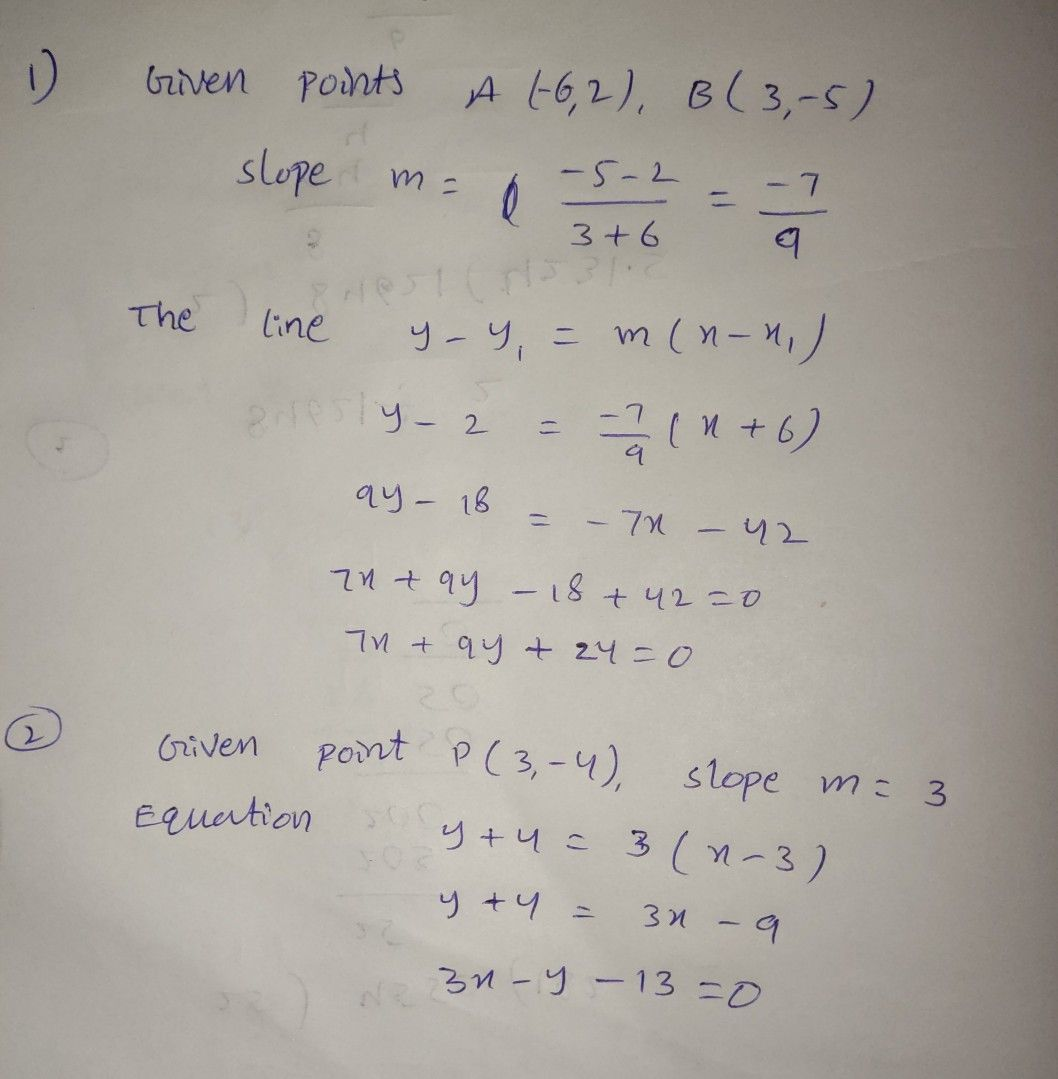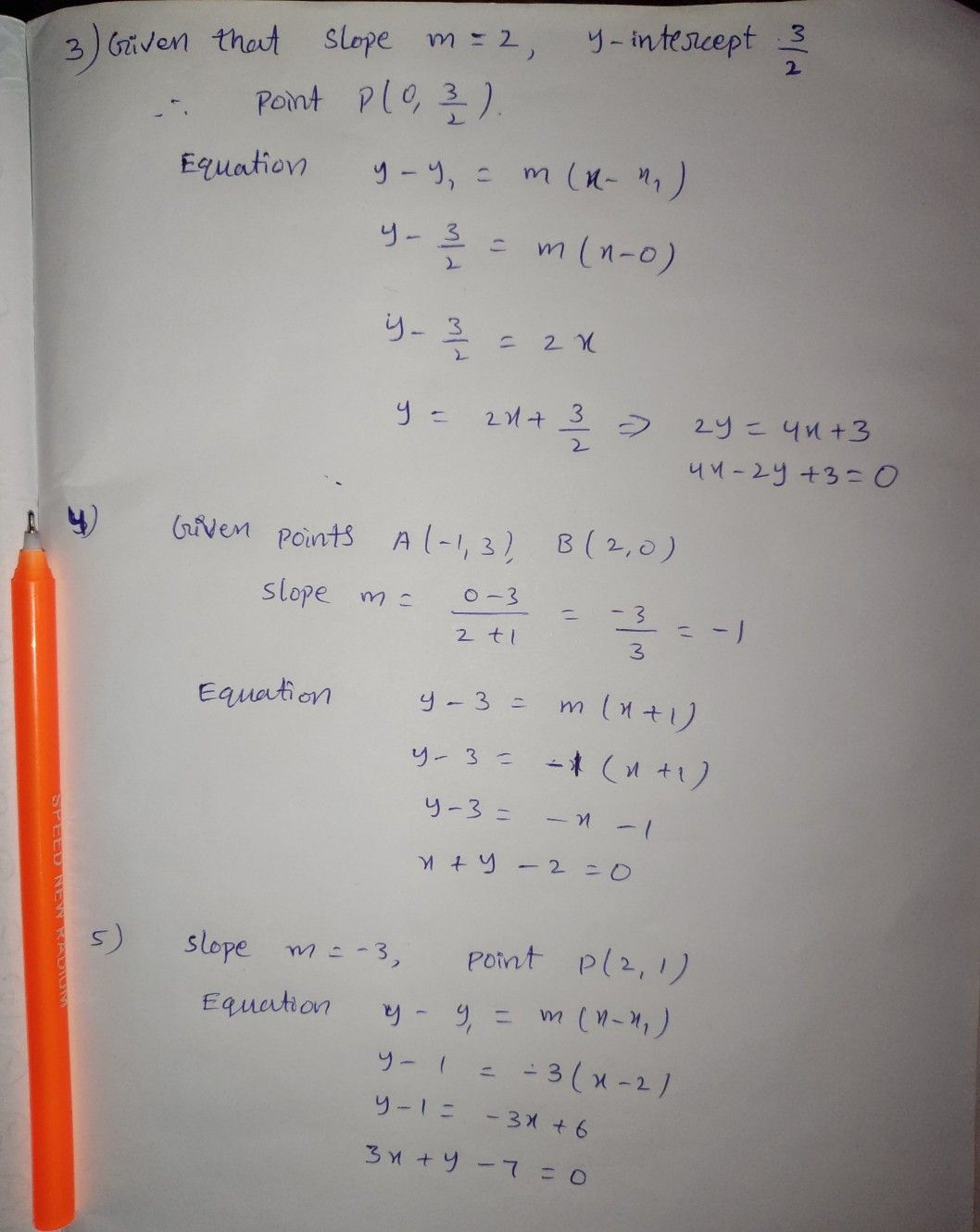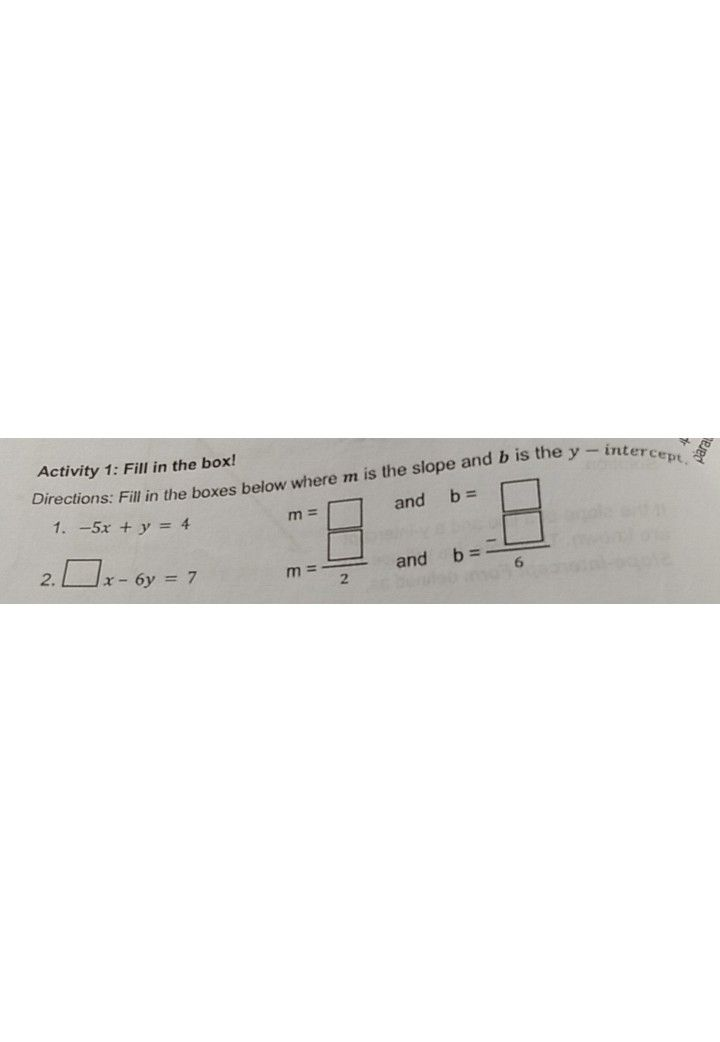Symbol
ProblemActivity 2: Let's Write an Equation! $Directions$ Write an equation of the line in $slope-$ intercept form given the $tollowin9$ $1.$ The line passes the points $\left(-6,2\right)$ and $\left(3,-5\right)$ $2.$ The line passes the point $\left(3,-4\right)$ and a slope of $3.$ $3.$ A line that has a slope of 2 and a $y-interc$ of $4$ The line passes through the points $\left(-1,3\right)$ and $\left(2$ $0\right)$ $5.$ The line has a slope $-3$ and passes through the point $\left(2,1\right)$